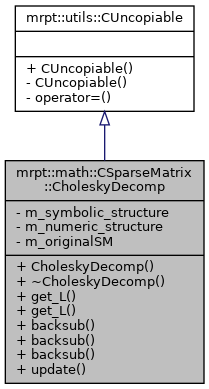Main MRPT website > C++ reference for MRPT 1.5.6
mrpt::math::CSparseMatrix::CholeskyDecomp Class Reference

Detailed Description

Auxiliary class to hold the results of a Cholesky factorization of a sparse matrix.

This implementation does not allow updating/downdating.

Usage example:

CSparseMatrix SM(100,100);
SM.insert_entry(i,j, val); ...
SM.compressFromTriplet();
// Do Cholesky decomposition:
CSparseMatrix::CholeskyDecomp CD(SM);
CD.get_inverse();
...
Note
Only the upper triangular part of the input matrix is accessed.
This class was initially adapted from "robotvision", by Hauke Strasdat, Steven Lovegrove and Andrew J. Davison. See http://www.openslam.org/robotvision.html
This class designed to be "uncopiable".
The main class: CSparseMatrix

Definition at line 360 of file CSparseMatrix.h.

`#include <mrpt/math/CSparseMatrix.h>`

Inheritance diagram for mrpt::math::CSparseMatrix::CholeskyDecomp:Public Member Functions

CholeskyDecomp (const CSparseMatrix &A)
Constructor from a square definite-positive sparse matrix A, which can be use to solve Ax=b The actual Cholesky decomposition takes places in this constructor. More...

virtual ~CholeskyDecomp ()
Destructor. More...

CMatrixDouble get_L () const
Return the L matrix (L*L' = M), as a dense matrix. More...

void get_L (CMatrixDouble &out_L) const
Return the L matrix (L*L' = M), as a dense matrix. More...

template<class VECTOR >
VECTOR backsub (const VECTOR &b) const
Return the vector from a back-substitution step that solves: Ux=b. More...

void backsub (const Eigen::VectorXd &b, Eigen::VectorXd &result_x) const
Return the vector from a back-substitution step that solves: Ux=b. More...

void backsub (const double *b, double *result, const size_t N) const
overload for double pointers which assume the user has reserved the output memory for result More...

void update (const CSparseMatrix &new_SM)
Update the Cholesky factorization from an updated vesion of the original input, square definite-positive sparse matrix. More...

Private Attributes

css * m_symbolic_structure

csn * m_numeric_structure

const CSparseMatrixm_originalSM
A const reference to the original matrix used to build this decomposition. More...

Constructor & Destructor Documentation

 CSparseMatrix::CholeskyDecomp::CholeskyDecomp ( const CSparseMatrix & SM )

Constructor from a square definite-positive sparse matrix A, which can be use to solve Ax=b The actual Cholesky decomposition takes places in this constructor.

Constructor from a square semidefinite-positive sparse matrix.

Note
Only the upper triangular part of the matrix is accessed.
Exceptions
 std::runtime_error On non-square input matrix. mrpt::math::CExceptionNotDefPos On non-definite-positive matrix as input.

The actual Cholesky decomposition takes places in this constructor.

Exceptions
 std::runtime_error On non-square input matrix. mrpt::math::CExceptionNotDefPos On non-semidefinite-positive matrix as input.

Definition at line 329 of file CSparseMatrix.cpp.

 CSparseMatrix::CholeskyDecomp::~CholeskyDecomp ( )
virtual

Destructor.

Definition at line 348 of file CSparseMatrix.cpp.

Member Function Documentation

template<class VECTOR >
 VECTOR mrpt::math::CSparseMatrix::CholeskyDecomp::backsub ( const VECTOR & b ) const
inline

Return the vector from a back-substitution step that solves: Ux=b.

Definition at line 387 of file CSparseMatrix.h.

References backsub().

Referenced by backsub().

 void CSparseMatrix::CholeskyDecomp::backsub ( const Eigen::VectorXd & b, Eigen::VectorXd & result_x ) const

Return the vector from a back-substitution step that solves: Ux=b.

Vectors can be Eigen::VectorXd or mrpt::math::CVectorDouble

Definition at line 362 of file CSparseMatrix.cpp.

References ASSERT_.

 void CSparseMatrix::CholeskyDecomp::backsub ( const double * b, double * result, const size_t N ) const

overload for double pointers which assume the user has reserved the output memory for result

Return the vector from a back-substitution step that solves: Ux=b.

Definition at line 372 of file CSparseMatrix.cpp.

References ASSERT_.

 CMatrixDouble mrpt::math::CSparseMatrix::CholeskyDecomp::get_L ( ) const
inline

Return the L matrix (L*L' = M), as a dense matrix.

Definition at line 380 of file CSparseMatrix.h.

References get_L().

Referenced by get_L(), and TEST().

 void CSparseMatrix::CholeskyDecomp::get_L ( CMatrixDouble & L ) const

Return the L matrix (L*L' = M), as a dense matrix.

Definition at line 355 of file CSparseMatrix.cpp.

References mrpt::math::CSparseMatrix::cs2dense().

 void CSparseMatrix::CholeskyDecomp::update ( const CSparseMatrix & new_SM )

Update the Cholesky factorization from an updated vesion of the original input, square definite-positive sparse matrix.

NOTE: This new matrix MUST HAVE exactly the same sparse structure than the original one.

Definition at line 393 of file CSparseMatrix.cpp.

References ASSERTMSG_, and mrpt::math::CSparseMatrix::sparse_matrix.

Member Data Documentation

 csn* mrpt::math::CSparseMatrix::CholeskyDecomp::m_numeric_structure
private

Definition at line 364 of file CSparseMatrix.h.

Referenced by CholeskyDecomp().

 const CSparseMatrix* mrpt::math::CSparseMatrix::CholeskyDecomp::m_originalSM
private

A const reference to the original matrix used to build this decomposition.

Definition at line 365 of file CSparseMatrix.h.

Referenced by CholeskyDecomp().

 css* mrpt::math::CSparseMatrix::CholeskyDecomp::m_symbolic_structure
private

Definition at line 363 of file CSparseMatrix.h.

Referenced by CholeskyDecomp().

 Page generated by Doxygen 1.8.6 for MRPT 1.5.6 Git: 4c65e84 Tue Apr 24 08:18:17 2018 +0200 at mar abr 24 08:26:17 CEST 2018